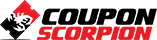# Complete Vedic Maths

The World’s Fastest Calculation System

### What you’ll learn

• Vedic Mathematics Give 10 -15 Times Faster Result.
• Increase your Speed and Accuracy.
• Develops Creativity And Confidence.
• Removes Maths phobia.
• You will start loving long maths calculations
• Solve Math Problem Right Away become a Math Genius

### Requirements

• Basic understanding of maths – nothing else!
• Book and Pen is required for practicing the problems

### Description

Would you like to solve bigger & bigger examples in just few seconds? Would you like to have fun with Math?

If yes then, This course is for you!

In this course you will learn all about High-Speed Vedic Mathematics and more.

This Course would help you to Learn amazing things to you, your kids/students which you might not have imagined.

You can multiply 9896 x 9997 in five seconds? Yes !!!

10 to 15 times faster than normal Math, Yes it is Worlds Fastest Calculation System

You will amaze to know that You too can solve

542372 X 999999 in just 6 Seconds

Cube Root of 373248 in just 2 Seconds

Cube of 32 in just 8 Seconds

Cube of 997 in just 15 Seconds

3217464    2014 in just 10 Seconds

This course will teach you secrets, which will make you capable of being human calculator.

Vedic math is the worlds fastest mental algebraic mathematics system. It was invented by Sri Bharati Krisna Tirthaji (1884-1960) and is still used today to calculate numbers rapidly. Vedic Mathematics is comprised of 16 wonderful sutras (math formulas) and 13 sub-sutras. The Vedic Maths presents a new approach to mathematics, offering simple, direct, one-line, mental solutions to mathematical problems.

Vedic math is the worlds fastest mental algebraic mathematics system

Topics Covered

In this course you will learn :-

• High Speed Multiplication

• Instant Subtractions

• Longer Division

• Digital Roots

• Algebra: Linear, Cubic, Quadratic equations

• Squares

• Cubes

• Square Roots

• Cube Roots

• Math Meditation – Solving Calculations without Pens and Paper!

• Divisibility

• Pythagoras Theorem

Learn the Power of Vedic Math in solving complex problems in few Seconds.

Author(s): Ismail Latkar

Deal Score+2
\$0 \$34.99